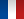• #### Number of hours

• Lectures 21.0
• Projects -
• Tutorials 21.0
• Internship -
• Laboratory works 8.0

ECTS 3.0

## Goal(s)

The objective of this module is to give the basics of fluid mechanics in three parts:

I Mechanics of fluids for the engineer.
II Navier-Stokes Equations
III Hydraulics of the flows in charge.

Responsible(s)

Thierry MAITRE, Regiane FORTES PATELLA

## Content(s)

I Mechanics of fluids for the engineer.
This section describes the tools needed to solve the problems of incompressible hydraulics without having recourse to the resolution of local equations of motion (Navier-Stokes). Hydrostatic, the laws of perfect fluid movement, mass balances, momentum and energy in real fluid are discussed successively.

II Navier-Stokes equation
This component leads to the general equations of the Navier-Stokes movement. The following are successively discussed the constraints (pressure and friction), the kinematics, the equations of motion. Application exercises of laminar flow equations are discussed. Finally, a final part concerns the adimensionalization of equations and the theory of similarity.

III Hydraulics of the flows in charge.
This component, organized around theoretical and experimental activities, aims to train engineering students in the apprenticeship sector on essential notions of flow hydraulics. The lessons will mainly concern the hydraulic circuits (mesh networks, calculation of pressure drops, design) and the transient regimes (hammer of mass and waves). The majority of the studies proposed will refer to concrete problems existing in the industry.

Prerequisites

Differential and integral calculus, vector analysis

Test

Exam, continuous evaluation with tests and practical works
Final mark for the module: 60% Exam and 40% continuous evaluation

EN 40% + ER 60%

Calendar

The course exists in the following branches:

• Curriculum - Master of Engineering GEE - Semester 5-6
see the course schedule for 2023-2024

Course language(s):